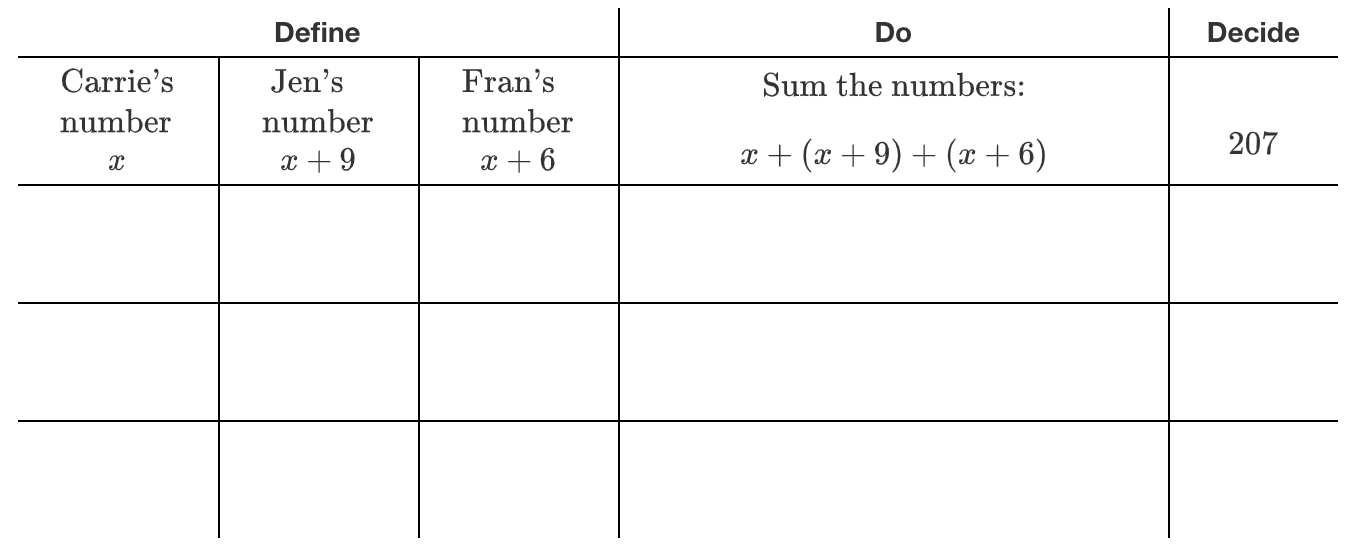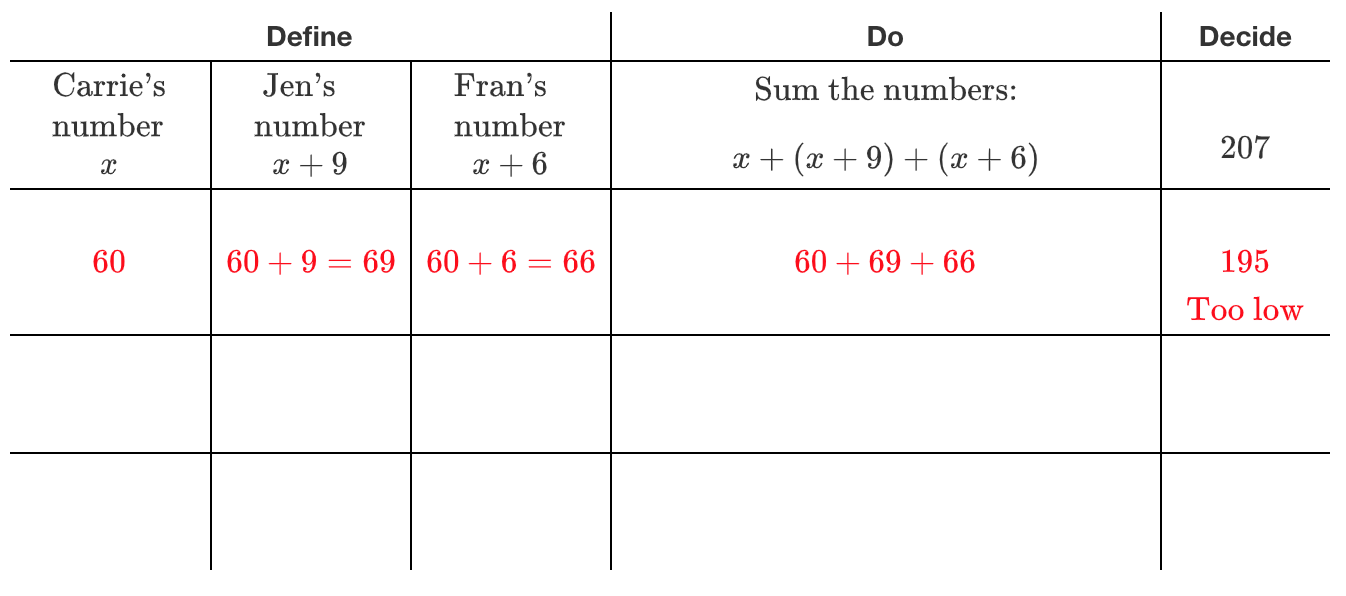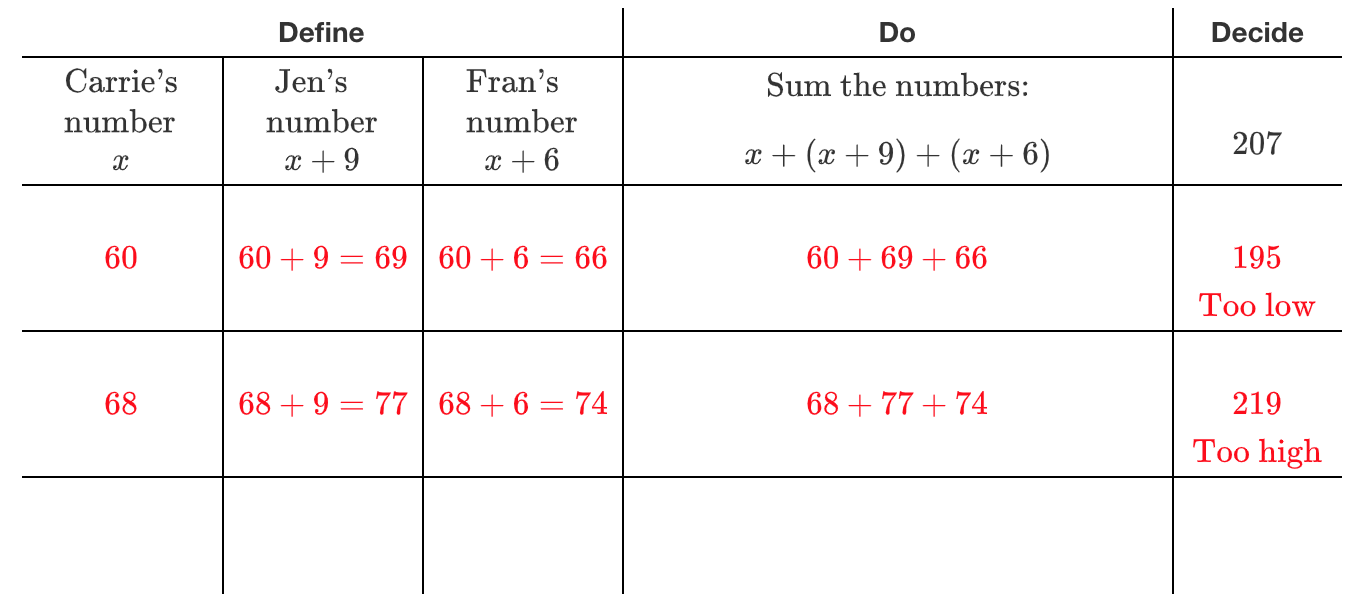### Home > CC3 > Chapter 8 > Lesson 8.1.3 > Problem8-33

8-33.

Write and solve an equation to solve the following problem. Be sure to define your variable and to state your answer in a complete sentence.

Jen, Carrie, and Fran are each thinking of a number. When you add their numbers together you get $207$. Jen’s number is $9$ more than Carrie’s, and Fran’s number is $3$ less than Jen’s number. What is Fran’s number?

For help writing an equation, start a 5-D table.

First row with 3 columns labeled: Define, do, and decide. Second row has 5 columns labeled as follows: the first 3 are under define: Carrie's number (x), Jen's number (x + 9), Fran's number (x + 6), Sum the numbers, (x) + (x + 9) + (x + 6), and 207.

Second row added labeled as follows: 60, 60 + 9 = 69, 60 + 6 = 66, 60 + 69 + 66, 195, too low.

Third row added labeled as follows: 68, 68 + 9 = 77, 68 + 6 = 74, 68 + 77 + 74, 219, too high.